International
Tables for
Crystallography
Volume B
Reciprocal space
Edited by U. Shmueli

International Tables for Crystallography (2010). Vol. B, ch. 2.5, pp. 379-381

## Section 2.5.7.6. 2D alignment of EM images

P. A. Penczekg

#### 2.5.7.6. 2D alignment of EM images

| top | pdf |

Alignment of pairs of 2D images is a fundamental step in single-particle reconstruction. It is aimed at bringing into register various particle projections by determining three orientation parameters (rotation angles and x and y translations) and is employed in 2D alignment of large sets of 2D noisy data and in 3D structure-refinement algorithms. The computational efficiency and numerical accuracy of this step are deciding factors in achieving high-quality structural results in an acceptable time.

All 2D alignment methods considered are aimed at finding transformation parameters such that the least-squares discrepancy between two images f and g is minimized,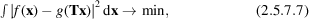where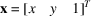is a vector containing the coordinates. T is the transformation matrix given by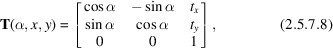and is dependent on three transformation parameters: rotation angle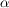and two translations tx and ty . It has to be noted that a minimum of (2.5.7.7)can be found rapidly using the fast Fourier transform (FFT) algorithm if only the xy translation is sought (2D FFT), or if only the rotation angle is needed (1D FFT).

2D alignment methods can be divided into three classes: (1) those that employ exhaustive searches in order to find three orientation parameters; (2) those that perform exhaustive searches by using either simplifications (separate searches for translation and rotation parameters) (Penczek et al., 1992) or by taking advantage of invariant image representations (Schatz & van Heel, 1990; Frank et al., 1992and the following discussion; Schatz & van Heel, 1992; Marabini & Carazo, 1996); or finally (3) those that are aimed at improvement of previously determined parameters and employ local searches.

In practice, as the windowed particles are approximately centred, the search for translation parameters can be restricted to relatively small values. A very efficient algorithm that takes advantage of the geometry is based on resampling to polar coordinates of the area of the image that roughly corresponds to the particle size. The resampling is done around centres placed on pixels located within a distance from the image centre that corresponds to a preset maximum translation (Joyeux & Penczek, 2002) (Fig. 2.5.7.2). For each translation, a 1D rotational cross-correlation function in polar coordinates is calculated. Overall, the alignment method based on resampling to polar coordinates comprises the following steps: (1) the image is resampled to polar coordinates; (2) 1D FFTs of various lengths are calculated, appropriately weighted and padded with zeros to equalize their lengths; (3) complex multiplications with 1D Fourier transforms of the similarly processed referenced image are calculated; (4) the inverse 1D FFT is calculated and the position of the maximum is found. The last step yields the rotation angle. Steps (1)–(4) are repeated with the image that is being aligned shifted to account for translations. In addition, the rotation angle for one of the images being mirrored is efficiently calculated in parallel with step (3) by repeating the multiplication with the 1D Fourier transforms of the reference image complex conjugated. This additional check is a necessity in the analysis of single-particle data sets, as usually one can expect on average half of the images to be mirrored versions of the other half in the data set. Overall, the method is very accurate, because only data under the circular mask enter the calculation.Figure 2.5.7.2 | top | pdf |The geometrical constraints of the 2D alignment problem. (a) The reference 2D particle is placed within a square image frame n × n pixels and its size is such that it can be bounded by a circle with a radius r no larger than 0.9n. (b) The particle projection, the size of which is bounded by the same radius as the reference view, can be located within a circle centred on discrete locations within the image frame, such that the maximum translation is k = (n/2) − r. The number of possible translations is (2k + 1)2. Reprinted from Joyeux & Penczek (2002)with permission from Elsevier.

For a set of N images containing the same object in various orientations and corrupted by an additive noise, the problem of alignment would be relatively simple. For proteins that have strong preferred orientation and particularly when a staining technique is used for grid preparation, this is certainly the case. In the procedure called reference-based alignment, one of the images that appears typical' is selected and used as a reference to align the remaining images. After all available images are aligned their average is calculated and used as a reference in a repeated alignment of all images. The process is iterated until the orientations of the images stabilize (Frank et al., 1982).

More formally, Frank et al. (1988) proposed the definition of a set of N images fk , k = 1, …, N, aligned if a set of transformations Tk, k = 1, …, N, (rotation angles and translations) is found such that all pairs of images are mutually brought into register, so the expression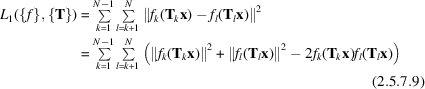is minimized. Although there is no simple way to minimize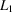, the interesting observation is that there is no requirement of the images to represent the same particle, not even a similar one. This leads to the conclusion that if the minimum ofcould be found, a set of diverse images could be aligned; moreover, upon alignment similar images would have similar orientation and subsequent classification of such an aligned data set would reveal subsets of similar images.

A practical method of minimizing, called a reference-free alignment, was proposed by Penczek et al. (1992) by showing that minimization ofis equivalent to maximization of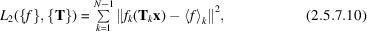where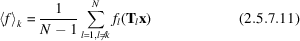is the partial average of the set of images calculated with the exclusion of the kth image. The method is based on the observation that given a set of approximately aligned images, it should be possible to minimize L2 by sequentially correcting alignments of individual images using the cross-correlation function between each image and the average of the remaining ones. On each step, depending whether the orientation of the image changes or not, (2.5.7.10)will decrease or remain constant.

The outcome of the reference-free alignment algorithm is an aligned set of N images, so all particles that have similar shapes will have similar orientations. Thus, it is natural (and because of the alignment possible) to divide the data set into classes of images that have similar shapes and orientations, i.e., to cluster them. A number of well known clustering algorithms have been adopted for EM applications (Frank, 1990). The general purpose of clustering is to organize objects (in the case of EM, images) into classes whose members are similar to each other, while dissimilar to objects from other classes.

Reference-free alignment with subsequent clustering works well as long as all particles share the same overall shape (i.e., the very low frequency component), as is the case for ribosomes. However, some molecules yield projections that have quite different shapes, as for example is the case for barrel-like proteins GroEL (Roseman et al., 1996) with rectangular views and circular end views or flat and rectangular hemocyanin (Boisset et al., 1995). In this case, the reference-free alignment tends to be unstable, as (2.5.7.10)has multiple local minima, which in practice means that the global average of the whole data set can vary significantly depending on the initiation of the procedure. In general, reference-free alignment is an alignment first, classification second' approach. It is possible to reverse this order by using invariants with the supporting rationale that once approximately homogeneous classes of images were found, it should be easy to align them subsequently as within each class they will share the same motif.

A practical approach to reference-free alignment known as alignment by classification (Dube et al., 1993) is based on the observation that for a very large data set and centred particles one can expect that although the in-plane rotation is arbitrary, there is a high chance that at least some of the similar images will be in the same rotational orientation. Therefore, in this approach the images are first (approximately) centred, then subjected to classification, and subsequently aligned.

In its simplest form, the multireference alignment belongs to the class of supervised classification methods: given a set of templates (i.e., reference images; these can be selected unprocessed particle projections, or class averages that resulted from preceding analysis, or projections of a previously determined EM structure, or projections of an X-ray crystallographic structure), each of the images from the available data sets is compared (using a selected discrepancy measure) with all templates and assigned to the class represented by the most similar one. Equally often multireference alignment is understood as a form of unsupervised classification, more precisely K-means classification, even if the description is not formalized in terms of the latter. Given a number of initial 2D templates, the images are compared with all templates and assigned to the most similar one. New templates are calculated by averaging images assigned to their predecessors and the whole procedure is repeated until a stable solution is reached.

### References

Boisset, N., Penczek, P., Taveau, J. C., Lamy, J. & Frank, J. (1995). Three-dimensional reconstruction of Androctonus australis hemocyanin labeled with a monoclonal Fab fragment. J. Struct. Biol. 115, 16–29.
Dube, P., Tavares, P., Lurz, R. & van Heel, M. (1993). The portal protein of bacteriophage SPP1: a DNA pump with 13-fold symmetry. EMBO J. 12, 1303–1309.
Frank, J. (1990). Classification of macromolecular assemblies studied as `single particles'. Quart. Rev. Biophys. 23, 281–329.
Frank, J., Penczek, P. & Liu, W. (1992). Alignment, classification, and three-dimensional reconstruction of single particles embedded in ice. Scan. Microsc. Suppl. 6, 11–20.
Frank, J., Radermacher, M., Wagenknecht, T. & Verschoor, A. (1988). Studying ribosome structure by electron microscopy and computer-image processing. Methods Enzymol. 164, 3–35.
Frank, J., Verschoor, A. & Boublik, M. (1982). Multivariate statistical analysis of ribosome electron micrographs. L and R lateral views of the 40 S subunit from HeLa cells. J. Mol. Biol. 161, 107–133.
Joyeux, L. & Penczek, P. A. (2002). Efficiency of 2D alignment methods. Ultramicroscopy, 92, 33–46.
Marabini, R. & Carazo, J. M. (1996). On a new computationally fast image invariant based on bispectral projections. Pattern Recognit. Lett. 17, 959–967.
Penczek, P., Radermacher, M. & Frank, J. (1992). Three-dimensional reconstruction of single particles embedded in ice. Ultramicroscopy, 40, 33–53.
Roseman, A. M., Chen, S., White, H., Braig, K. & Saibil, H. R. (1996). The chaperonin ATPase cycle: mechanism of allosteric switching and movements of substrate-binding domains in GroEL. Cell, 87, 241–251.
Schatz, M. & van Heel, M. (1990). Invariant classification of molecular views in electron micrographs. Ultramicroscopy, 32, 255–264.
Schatz, M. & van Heel, M. (1992). Invariant recognition of molecular projections in vitreous ice preparations. Ultramicroscopy, 45, 15–22.